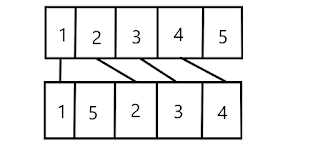# Minimum steps to transform first permuation into second permutation

## Problem Statement

Given an integer N and two permutation of length N(1 through N ),the task is to convert first permutation to second permutation by following operations:

• Select the last number of first permutation
• Insert it back in the first permutation at any arbitrary (except last position)

Determine the minimum number of transformations required to convert first permutation to second permutation

Example:

input:

N=5

A=[1,2,3,4,5] B=[1,5,2,3,4]

output:

explanation:Insert 5 between 1&2,First permutation becomes->[1,5,2,3,4]

input:N=3 A=[3,2,1] B=[1,2,3]

output:2

Approach:if the topological order (sequence relationship) of the first i (continuous) elements of first permutation meets the target replacement (requirements i.e sub sequence of second permutation ), then the answer must be less than or equal to n-i,Because the last n-i elements can be selected optimally & inserted in its proper position, and will not be selected the second time.Therefore to solve the problem, we can find the length of first i continuous elements (1≤i≤N & i should start from 1st index) of first permutation which is present as sub sequence in the second permutation & then subtracting that length from the original length of the array.

illustration 1:Sequence

In the above example the first four (continuous) elements of the first permutation i.e(1,2,3,4) of length-4, forms sub sequence in second permutation. i.e it is in sequence relationship with the target permutation which means that the inserting (N-i) elements optimally will give us the desired result.

So i=4 & operations needed =(n-i)=>(5-4)=1.

illustration 2:Sequence

In the above example only the first two (continuous) elements i.e(1 & 5) of first permutation forms sub sequence in second permutation.

So i=2 & operations needed =(n-i)=>(5-2)=3.

## Solution

Below is the Java implementation of the above approach:

Code

import java.io.*;

class Solution {
public static int minCount(int[] a, int[] b, int n)
{
int i = 0;
for (int j = 0; j < n; j++) {
// if element of A
// at position j is equal
// to element of B at position i
// then increase j by 1
if (a[i] == b[j]) {
i++;
}
}
return n - i;
}
// driver code
public static void main(String[] args)
{
int n = 5;
int[] a = { 1, 2, 3, 4, 5 };
int[] b = { 1, 5, 2, 3, 4 };
// function calling
System.out.println(minCount(a, b, n));
}
}

Output:

1

Time Complexity:O(n)

Auxiliary Space:O(1)

Feel free to comment if you have any doubt.

For more Data structure and algorithms,computer science,programming,coding related problems search my website.

Learn how to prepare for Information Technology Based companies here